# Colligative Properties Relative Lowering Of Vapour Pressure

## Derivation of Roults law and Relative lowering of Vapour Pressure

Colligative Properties Relative Lowering of Vapour Pressure

The colligative properties can be defined as the properties of solutions which is wholly determined by the ratio of the number of solute particles and the number of solvent molecules in a particular solution, and are completely independent of the nature of the chemical species present. The number ratio can be calculated by using various units that determines the concentration of solutions. The general assumption is that in case of an ideal solution the properties are independent of the nature of solute particles present in it and is somewhat approximate for dilute real solutions. In other words, colligative properties can be considered as a set of properties of the solution that can be reasonably approached if we follow the assumption that the solution is ideal.

Here we have considered only those properties which are formed from the dissolution of a non-volatile solute in a volatile liquid solvent. Essentially the solvent properties are changed due to the presence of the solute. The solute particles actually displace some solvent molecules in the liquid phase and thus resulting in the reduction of the concentration of solvent. Thus we may conclude that the colligative properties are independent of the nature of the solute. The word colligative has been derived from the Latin colligatus which means bound together.

Colligative properties of a solution include:

• • Relative lowering of vapor pressure

• • Elevation of boiling point

• • Depression of freezing point

• • Osmotic pressure

• For a particular mass ratio of solute and solvent, all colligative properties should be inversely proportional to solute molar mass.

Relative molar masses can be determined by measuring colligative properties for a dilute solution of a non-ionized solute. Such solutions include urea or glucose in water or another solvent. This is applicable for both small molecules and for polymers. In an alternate manner, the percentage of dissociation taking place can be estimated by measuring ionized solutes.

Colligative properties are mostly applicable for dilute solutions as their behavior may often be approximated. This is because they are ideal solutions.

This unit will focus mainly on the relative lowering of vapor pressure.

RELATIVE LOWERING OF VAPOR PRESSURE

On dissolving the non-volatile solute in a pure solvent, the vapor pressure of a pure solvent gets gradually decreased
Let us assume that p is the vapor pressure of the solvent and psis the vapor pressure of the solution, then the lowering of vapor pressure can be written as (p – ps). This lowering of vapor pressure relative to the vapor pressure of the pure solvent is called the Relative lowering of Vapour pressure. Thus,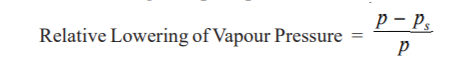After extensive experimentation, Raoult (1886) gave an empirical relation to establishing the connection between the
relative lowering of vapor pressure and the concentration of the solute in a solution. This is now referred to as the Raoult's Law. As per this law, the relative lowering of the vapor pressure of a dilute solution is equal to the mole fraction of the solute present in dilute solution.

Mathematically Raoult’s Law can be expressed in the form :
where n = number of moles or molecules of solute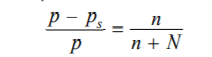Derivation of Raoult's law

The vapor pressure of the pure solvent is the result caused by the number of molecules evaporating from its surface. When a non-volatile solute is dissolved in solution, due to the presence of solute molecules in the surface a fraction of the surface gets blocked and here no evaporation can take place.

By Lowering of vapor pressure by a non-volatile solute the particles of the solute prohibit the escape of solvent molecules from the surface of the solution. This finally results in the lowering of the vapor pressure. The vapor pressure of the solution is, therefore, dependent on the number of molecules of the solvent found at any time in the surface which is again proportional to the mole fraction. That is,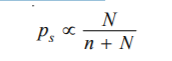where N = moles of solvent and n = moles of solute.
Or we can write it in the form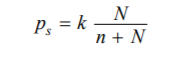k being proportionality factor.
In case of pure solvent n = 0 and hence Mole fraction of solvent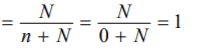Now from equation (1), the vapor pressure p = k Therefore the equation (1) assumes the form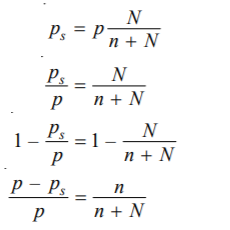This is Raoult’s law.

Ideal Solutions and Deviations from Raoult’s Law

A solution which strictly follows the Raoult’s law strictly is called an ideal solution. A solution which shows even slight deviations from Raoult’s law is called a non-ideal or Real solution. Let us consider that the molecules of the solvent and solute are represented by A and B respectively. Now let γAB be the representation of the attractive force acting between A and B, and γAA between A and A. If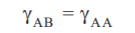the solution will have the same vapor pressure as predicted by Raoult's law and it is an ideal solution. However, if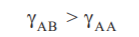molecule A will escape comparatively less readily and the vapor pressure will then be less than that of the predicted one, which is calculated by obeying Raoult’s law (Such deviation is called Negative deviation). On the other hand, if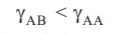A molecule will get to escape from the solution surface more rapidly and then the vapor pressure of the solution will become higher than predicted by Raoult’s law (Such deviation is then called the Positive deviation). If we consider very dilute solutions of nonelectrolytes, the solvent and solute molecules are very much similar be it in terms of molecular size or be its molecular attractions. Thus, under such situations, the given solutions have the tendency to approach the ideal behavior and obey
Raoult's law more or less accurately.

Determination of Molecular Mass from Vapour Pressure Lowering

The molecular mass of a non-volatile solute can be calculated from the measurement of the lowering of vapor pressure (p – ps ) produced by dissolving a known weight of it in a known weight of the solvent. It is considered that w grams of solute is dissolved in W grams of the solvent, and let m and M are molecular masses of the solute and solvent respectively, we have :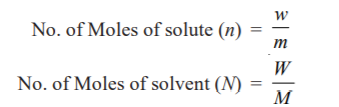Substituting these values in Raoult’s law Equation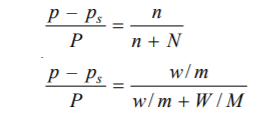Considering an extremely diluted solution, the number of moles (molecules) of solute (w/m), is very very small, it can be then neglected in the denominator. The equation (1) can then be written as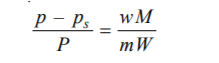With the known experimental value of p – ps /p, and the molecular mass of the solvent (M), the molecular weight of solute (m) can be calculated from the above equations.

MEASUREMENT OF LOWERING OF VAPOUR PRESSURE

• 1. BarometricMethod: The individual vapor pressure of a liquid was calculated by Raoult and then the same process was followed to calculate the vapor pressure of the solution as well. He poured the liquid or the solution into Toricellian vacuum of a barometer tube and calculated the depression of the mercury level. This method was later found to be neither practicable nor accurate as the lowering of vapor pressure is almost negligible.

• 2. Manometric method: The vapor pressure of a liquid or solution can be fairly measured with the help of a manometer. Let us assume a bulb is charged with the liquid or solution. The air in the connecting tube of the instrument is then removed with a vacuum pump. With the stopcock being closed, the pressure inside is only due to the vapor evaporating from the solution or liquid. This method can be applied to aqueous solutions. The manometric liquid used can be either mercury or n-butyl phthalate which has low density and low volatility.

• 3. Ostwald and Walker’s Dynamic Method (Gas Saturation Method): In this method, the relative lowering of vapor pressure can be calculated in an easy simple way Procedure. The apparatus used by Ostwald and Walker consists of two sets of bulbs : (a) Set A contains the solution (b) Set B contains the solvent. The weight of each set is calculated separately. A slow stream of dry air is then removed by a suction pump through the two sets of bulbs. At the end of the operation, the weight of these sets is again measured. From the weight loss in each of the two sets, the lowering of vapor pressure is measured. But here the temperature of the air, the solution, and the solvent must be kept constant all throughout. As the air bubbles through set A reaches saturation up to the vapor pressure ps of the solution and then up to vapor pressure p of solvent in set B, the amount of solvent taken up in set A becomes proportional to ps and the amount taken up in set B becomes proportional to (p – ps ).

• Ostwald-Walker method of measuring the relative lowering of vapor pressure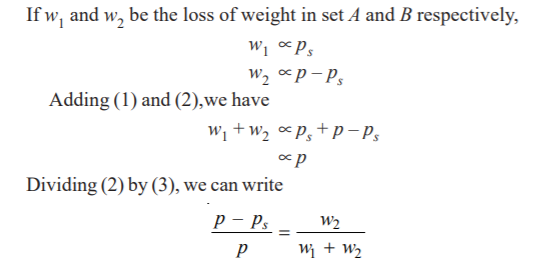Knowing the loss of mass in set B (w2) and the net loss of mass in the two sets (w1 + w2), we can find the relative lowering of vapor pressure. If we use water as the solvent, a set of calcium chloride tubes (or a set of bulbs containing conc. H2SO4) is linked to the end of the apparatus to capture the escaping water vapor. Therefore, the gain in mass of the CaCl2-tubes will be equal to (w1 + w2), the total loss of mass in sets A and B.